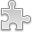# Courses / Module

Toggle Print

##ORDINARY DIFFERENTIAL EQUATIONS

Module code: MT301C
Credits: 5
Semester: 2
Department: MATHEMATICS AND STATISTICS
International:Overview

Module Objective:
To introduce students to Ordinary Differential Equations and Modelling.

First Order Equations: separable, homogeneous, linear, Bernoulli. Second Order Equations: constant coefficient, Euler type, linear equations. Methods of solution including reduction of order, variation of parameters, undetermined coefficients. Miscellaneous Equations of special type.

Modelling of applied problems such as the spread of infections, radioactive decay, population growth, Newton's Law of Cooling.
Computation using mathematical/statistical software.Learning OutcomesTeaching & Learning methodsAssessmentAutumn Supplementals/ResitsPre-RequisitesTimetable